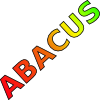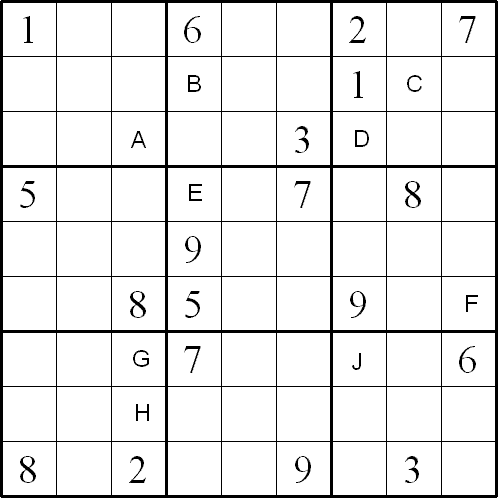#### You may also like### Consecutive Numbers

An investigation involving adding and subtracting sets of consecutive numbers. Lots to find out, lots to explore.### Tea Cups

Place the 16 different combinations of cup/saucer in this 4 by 4 arrangement so that no row or column contains more than one cup or saucer of the same colour.### Counting on Letters

The letters of the word ABACUS have been arranged in the shape of a triangle. How many different ways can you find to read the word ABACUS from this triangular pattern?

# Alphabetti Sudoku

##### Age 11 to 16Challenge Level

By Henry Kwok#### Rules of "Alphabetti Sudoku"

Like the standard sudoku, this sudoku variant consists of a grid of nine rows and nine columns subdivided into nine 3x3 subgrids.
It has two basic rules:
• Each column, each row, and each box (3x3 subgrid) must have the numbers 1 through 9
• No column, row or box can have two squares with the same number.

The puzzle can be solved with the help of some starting digits and 9 upper-case letters in certain squares.
The 9 upper-case letters (J and A to H) stand for the 9 mathematical problems given below.
Find the digit in each box of the given problems. After that, you will have to substitute all the letters with the answers and solve the sudoku puzzle with the usual strategy and techniques.

Below are the nine problems. Can you find the answer in each of the problems?

$A. \; 631 - 2\bigcirc 9 = 372$
$B. \; 5\bigcirc 4 + 584 = 1128$
$C. \; 318 \times 6\bigcirc 3 = 220374$
$D. \; 140781 \div 1\bigcirc 7 = 843$
$E. \; 13\bigcirc :209 = 7:11$
$F. \; 32\%$ of $7\bigcirc 1 = 230.72$
$G. \; \frac{\bigcirc 2}{45} \times \frac{5}{4} = \frac{1}{3}$
$H. \; \frac{3}{5} - \frac{\bigcirc}{12} = \frac{1}{60}$
$J. \;$The average of $98$ and $\bigcirc 6$ is $92.$